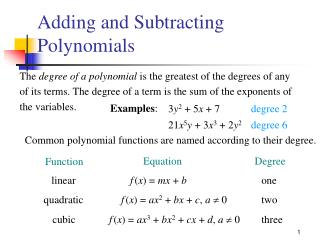DownloadDownload PresentationTélécharger la présentation- - - - - - - - - - - - - - - - - - - - - - - - - - - E N D - - - - - - - - - - - - - - - - - - - - - - - - - - -
Presentation Transcript

1. Equation Degree Function linear f(x) = mx + b one quadratic f(x) = ax2 + bx + c, a 0 two cubic f(x) = ax3 + bx2 + cx + d, a 0 three Adding and Subtracting Polynomials The degree of a polynomial is the greatest of the degrees of any of its terms. The degree of a term is the sum of the exponents of the variables. Examples: 3y2 + 5x +7 degree 2 21x5y + 3x3+2y2 degree 6 Common polynomial functions are named according to their degree.

2. Adding and Subtracting Polynomials To add polynomials, combine like terms. Examples: Add (5x3 + 6x2 + 3) + (3x3 – 12x2 – 10). Use a horizontal format. Rearrange and group like terms. (5x3 + 6x2 + 3) + (3x3 – 12x2 – 10) = (5x3 + 3x3 ) + (6x2 – 12x2) + (3 – 10) = 8x3 – 6x2 – 7 Combine like terms.

3. Adding and Subtracting Polynomials Add (6x3 + 11x –21) + (2x3 + 10 – 3x) + (5x3 + x – 7x2 + 5). Use a vertical format. 6x3 + 11x – 21 Arrange terms of each polynomial in descending order with like terms in the same column. 2x3 – 3x + 10 5x3 – 7x2 + x + 5 13x3 – 7x2 + 9x – 6 Add the terms of each column.

4. Adding and Subtracting Polynomials The additive inverse of the polynomial x2 + 3x + 2 is –(x2 + 3x + 2). This is equivalent to the additive inverse of each of the terms. –(x2 + 3x + 2) = – x2 – 3x – 2 To subtract two polynomials, add the additive inverse of the second polynomial to the first.

5. Adding and Subtracting Polynomials Example: Add (4x2 – 5xy + 2y2) – (–x2 + 2xy – y2). (4x2 – 5xy + 2y2) – (–x2 + 2xy – y2) Rewrite the subtraction as theaddition of the additive inverse. = (4x2 – 5xy + 2y2) + (x2 – 2xy + y2) = (4x2 + x2) + (–5xy – 2xy) + (2y2 + y2) Rearrange and group like terms. = 5x2 – 7xy + 3y2 Combine like terms.

6. Multiplying Polynomials To multiply a polynomial by a monomial, use the distributive property and the rule for multiplying exponential expressions. Examples:. Multiply:2x(3x2 + 2x – 1). = 2x(3x2) + 2x(2x)+ 2x(–1) = 6x3 + 4x2 – 2x

7. Multiplying Polynomials Multiply:–3x2y(5x2 – 2xy + 7y2). = –3x2y(5x2) – 3x2y(–2xy) – 3x2y(7y2) = –15x4y + 6x3y2 – 21x2y3

8. Multiplying Polynomials To multiply two polynomials, apply the distributive property. Don’t forget to apply properties of exponents!!! Example: Multiply: (x – 1)(2x2 + 7x + 3). = (x – 1)(2x2) + (x – 1)(7x) + (x – 1)(3) = 2x3 – 2x2 + 7x2 – 7x + 3x – 3 = 2x3 + 5x2 – 4x – 3

9. Example: 2x2 + 7x + 3 x – 1 Multiplying Polynomials Example: Multiply: (x – 1)(2x2 + 7x + 3). Two polynomials can also be multiplied using a vertical format. – 2x2 – 7x – 3 Multiply –1(2x2 + 7x + 3). 2x3 + 7x2 + 3x Multiply x(2x2 + 7x + 3). Add the terms in each column. 2x3 + 5x2 – 4x – 3

10. Multiplying Polynomials To multiply two binomials use a method called FOIL, which is based on the distributive property. The letters of FOIL stand for First, Outer, Inner, and Last. 1. Multiply the first terms. 4. Multiply the last terms. 2. Multiply the outer terms. 5. Add the products. 3. Multiply the inner terms. 6. Combine like terms.

11. Last Outer Inner First Multiplying Polynomials Examples: Multiply:(2x +1)(7x – 5). = 2x(7x) + 2x(–5) + (1)(7x) + (1)(–5) = 14x2 – 10x + 7x – 5 = 14x2 – 3x – 5

12. First Last Outer Inner Multiplying Polynomials Multiply:(5x – 3y)(7x + 6y). = 5x(7x) + 5x(6y) + (– 3y)(7x) + (– 3y)(6y) = 35x2 + 30xy – 21yx – 18y2 = 35x2 + 9xy – 18y2

13. Special Cases for Multiplication The multiply the sum and difference of two terms, use this pattern: (a + b)(a – b) = a2 – ab + ab – b2 = a2 – b2 square of the second term square of the first term

14. Special Cases for Multiplication Examples: (3x + 2)(3x – 2) (x + 1)(x – 1) = (3x)2– (2)2 = (x)2– (1)2 = 9x2– 4 = x2– 1

15. Special Cases for Multiplication To square a binomial, use this pattern: (a + b)2 = (a + b)(a + b) = a2 + ab + ab + b2 = a2 + 2ab + b2 square of the first term twice the product of the two terms square of the last term

16. Special Cases for Multiplication Examples: Multiply: (2x – 2)2 . = (2x)2 + 2(2x)(– 2) +(– 2)2 = 4x2 – 8x + 4 Multiply: (x + 3y)2 . = (x)2 + 2(x)(3y) +(3y)2 = x2 + 6xy + 9y2

17. x – 6 x + 5 Example: The length of a rectangle is (x + 5) ft. The width is (x – 6) ft. Find the area of the rectangle in terms of the variable x. A = L · W = Area L = (x + 5) ft W = (x – 6) ft A = (x + 5)(x – 6 ) = x2 – 6x + 5x – 30 = x2 – x – 30 The area is (x2 – x – 30) ft2.